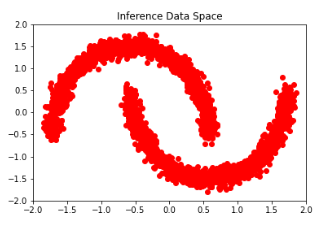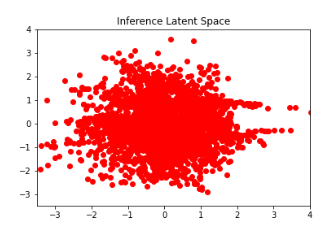#keras-io /real_nvp

## Model description

This model demonstrates real-valued non-volume preserving (real NVP) transformations, a tractable yet expressive approach to modeling high-dimensional data.

This model is used to map a simple distribution - which is easy to sample and whose density is simple to estimate - to a more complex one learned from the data. This kind of generative model is also known as "normalizing flow". The latent distribution we wish to map to in this example is Gaussian.

## Training and evaluation data

This model is trained on a toy dataset, the make_moons from sklearn.datasets.

## Requirements

This model requires

• Tensorflow 2.9.1
• Tensorflow probability 0.17.0

Only the model weights are stored. To load this model, use load_model function from load_model.py.

## Original Data Distribution and Latent Distribution### Training hyperparameters

The following hyperparameters were used during training:

name learning_rate decay beta_1 beta_2 epsilon amsgrad training_precision
Adam 9.999999747378752e-05 0.0 0.8999999761581421 0.9990000128746033 1e-07 False float32